HOTS Questions: Quadratic Equations

# HOTS Questions: Quadratic Equations Notes | Study Mathematics (Maths) Class 10 - Class 10

## Document Description: HOTS Questions: Quadratic Equations for Class 10 2022 is part of Mathematics (Maths) Class 10 preparation. The notes and questions for HOTS Questions: Quadratic Equations have been prepared according to the Class 10 exam syllabus. Information about HOTS Questions: Quadratic Equations covers topics like and HOTS Questions: Quadratic Equations Example, for Class 10 2022 Exam. Find important definitions, questions, notes, meanings, examples, exercises and tests below for HOTS Questions: Quadratic Equations.

Introduction of HOTS Questions: Quadratic Equations in English is available as part of our Mathematics (Maths) Class 10 for Class 10 & HOTS Questions: Quadratic Equations in Hindi for Mathematics (Maths) Class 10 course. Download more important topics related with notes, lectures and mock test series for Class 10 Exam by signing up for free. Class 10: HOTS Questions: Quadratic Equations Notes | Study Mathematics (Maths) Class 10 - Class 10
 1 Crore+ students have signed up on EduRev. Have you?

Q1. Had Ravita scored 10 more marks in her Mathematics test out of 30 marks, 9 times these marks would have been the square of her actual marks. How many marks did she get in the test?

Sol. Let actual marks be x
∴ 9 × [ Actual marks + 10] = [Square of actual marks]
or 9 × (x + 10) = x2
⇒ 9x + 90 = x2
⇒ x2 – 9x – 90 = 0
⇒ x2 – 15x + 6x – 90 = 0
⇒ x(x – 15) + 6(x – 15) = 0
⇒(x + 6) (x – 15) = 0
Either  x + 6 =  0
⇒ x = – 6 or x – 15 = 0
⇒ x = 15
But marks cannot be less than 0.
∴ x = –6 is not desired.

Thus, Ravita got 15 marks in her Mathematics test.

Q2. A motor boat whose speed is 18 km/h in still water takes 1 hour more to go 24 km upstream than to return downstream to the same spot. Find the speed of the stream.

Sol. Let the speed of the stream = x km/hr
∴ speed of the motor boat:
upstream = (18 − x) km/hr
downstream = (18 + x) km/hr
⇒ Time taken by the motor boat in going: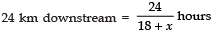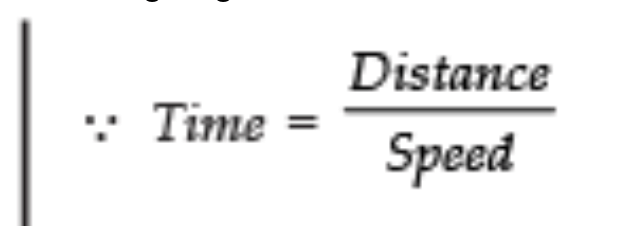24 km upstream = 20/18-x hours

According to the condition: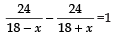⇒ 24 × (18 + x) − 2(18 − x) = 1 (18 − x) (18 + x)
⇒ 24 [18 + x − 18 + x] = 182 − x2
⇒ 24 [2x] = 324 − x2
⇒ 48x = 324 − x2
⇒ x2 + 48x − 324 = 0
⇒ x2 + 54x − 6x − 324 = 0
⇒ x (x + 54) − 6 (x + 54) = 0
⇒ (x − 6) (x + 54) = 0
Either x − 6 = 0 ⇒ x = 6
or x + 54  = 0 ⇒ x = − 54

But speed cannot be negative
∴ Rejecting x =− 54, we have

x = 6 ⇒ Speed of the boat = 6 km/hr.

Q3. In a class test, the sum of marks obtained by P in Mathematics and Science is 28. Had he got 3 more marks in Mathematics and 4 marks less in Science, the product of marks obtained in the two subjects would have been 180? Find the marks obtained in two subjects separately.

Sol. Let marks obtained by P in Maths be ‘x’.
∴ His marks in Science = (28 − x)
According to the condition,

(x + 3) (28 − x − 4) = 180
⇒ (x + 3) (− x + 24) = 180
⇒ 24x − x2 + 72 − 3x = 180
⇒ − x2 + 21x + 72 − 180 = 0
⇒ − x2 + 21x − 108 = 0
⇒ x2 − 21x + 108 = 0
⇒ x2 − 12x − 9x + 108 = 0
⇒ x (x − 12x) − 9(x − 12) = 0
⇒ (x − 9) (x − 12) = 0
⇒ (x − 9) (x − 12) = 0

Either  x − 9 = 0
⇒ x = 9 or  x − 12 = 0
⇒ x = 12 When x = 9 then 28 − x = 28 − 9 = 19
When x = 12 then 28 − x = 28 − 12 = 16
Thus P’s marks in Maths = 9 and Science = 19

Or

P’s marks in Maths = 12 and Science = 16.

Q4. At ‘t’ minutes past 2 pm, the time needed by the minute hand of a clock to show 3 pm was found to be 3 minutes less than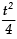minutes. Find ‘t’.

Sol. For a minute-hand time needed to show 2 pm to 3 pm is ‘60’ minutes. It has already covered ‘t’ minutes. ∴ Time required by the minute-hand to reach to 12 (at 3 pm) = (60 – t) minutes.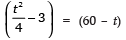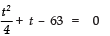⇒ t2+ 4t – 252 = 0

Solving, we get,  t = 14 or – 18
But t = – 18 is not desirable (being negative)
Thus, t = 14 minutes.

Q5. A train, travelling at a uniform speed for 360 km, would have taken 48 minutes less to travel the same distance if its speed were 5 km/ hr more. Find the original speed of the train.

Sol. Let the original speed be x km/hr

∴ Original time taken = 360/x.

New speed = (x + 5) km/hr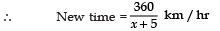According to the condition,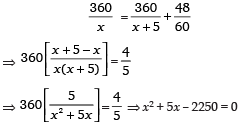Solving for x, we get x = – 50 or 45
Speed cannot be negative
∴ Rejecting x = – 50, we have x = 45
Thus, the original speed of the train = 45 km/hr.

The document HOTS Questions: Quadratic Equations Notes | Study Mathematics (Maths) Class 10 - Class 10 is a part of the Class 10 Course Mathematics (Maths) Class 10.
All you need of Class 10 at this link: Class 10

## Mathematics (Maths) Class 10

53 videos|369 docs|138 tests
 Use Code STAYHOME200 and get INR 200 additional OFF

## Mathematics (Maths) Class 10

53 videos|369 docs|138 tests

Track your progress, build streaks, highlight & save important lessons and more!

,

,

,

,

,

,

,

,

,

,

,

,

,

,

,

,

,

,

,

,

,

;